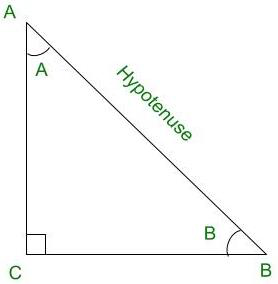# If 2y cos θ = x sin θ and 2x sec θ – y csc θ = 3, then prove that x2 + 4y2 = 4

• Last Updated : 21 Sep, 2021

Trigonometry is the relation between the angles and sides of a right-angled triangle. In a right angles triangle, there are 3 angles of which one angle is a right angle (90°) and the other two angles are acute angles and there are 3 sides. The side opposite to the right angle is called Hypotenuse. There are 6 ratios between these sides based on the angle between them and they are called Trigonometric Ratios.

The 6 Trigonometric ratios are:

Attention reader! All those who say programming isn't for kids, just haven't met the right mentors yet. Join the  Demo Class for First Step to Coding Coursespecifically designed for students of class 8 to 12.

The students will get to learn more about the world of programming in these free classes which will definitely help them in making a wise career choice in the future.

• Sine (sin)
• Cosine (cos)
• Tangent (tan)
• Cosecant (cosec)
• Secant (sec)
• Cotangent (cot)Right-angled triangle ACB

Sine (sin):

Sine of an angle is defined by the ratio of lengths of sides which is opposite to the angle and the hypotenuse. For the above triangle, the value of angle sine is given for both ∠A and ∠B, the definition for sine angle is the ratio of the perpendicular to its hypotenuse.Cosine (cos):

Cosine of an angle is defined by the ratio of lengths of sides which is adjacent to the angle and the hypotenuse. For the above triangle, the value of the angle cos is given for both ∠A and ∠B, the definition for cos angle is the ratio of the base to its hypotenuse.Tangent (tan):

The tangent of an angle is defined by the ratio of the length of sides which is opposite to the angle and the side which is adjacent to the angle. For the above triangle, the value of the angle tan is given for both ∠A and ∠B, the definition for tan angle is the ratio of the perpendicular to its base.Cosecant (cosec):

The cosecant of an angle is defined by the ratio of the length of the hypotenuse and the side opposite the angle. For the above triangle, the value of the angle cosec is given for both ∠A and ∠B, the definition for cosec angle is the ratio of the hypotenuse to its perpendicular.Secant (sec):

Secant of an angle is defined by the ratio of the length of the hypotenuse and the side and the side adjacent to the angle. For the above triangle, the value of the angle sec is given for both ∠A and ∠B, the definition for sec angle is the ratio of the hypotenuse to its base.Cotangent (cot):

The cotangent of an angle is defined by the ratio of the length of sides that is adjacent to the angle and the side which is opposite to the angle. For the above triangle, the value of the angle cot is given for both ∠A and ∠B, the definition for cot angle is the ratio of the hypotenuse to its base.### If 2y cosθ= x sinθand 2x secθ- y cosecθ= 3, then prove that x2 + 4y2 = 4

Solution:

2ycosθ= xsinθ …….. (1)2ycosecθ= xsecθ …….. (2)

2xsecθ− ycosecθ= 3

From (2), we get

2(2ycosecθ) − ycosecθ= 34ycosecθ− ycosecθ= 33ycosecθ= 3ycosecθ= 1y =y = sinθ …….. (3)

From (1), we get

2ycosθ= xsinθ

2sinθcosθ= xsinθ …….. (From (3))cosθ=……… (4)

We know that sin2θ+ cos2θ= 1y2 += 1 ………(From (3) and (4))

Multiplying by 4 on both sides;

x2 + 4y2 = 4

Hence, Proved

### Similar Problems

Question 1: If xcosθ – ysinθ = √(x2 + y2) and, then+= 1

Solution:

xcosθ – ysinθ = √(x2 + y2)…… (1)

We know that sin2θ + cos2θ = 1 ⇒ sinθ.sinθ + cosθ.cosθ = 1 …… (2)

Comparing equations (1) and (2), we get,

sinθ =cosθ =It is given that,Hence, Proved.

Question 2: If asinθ – bcosθ = c, then prove that, acosθ + bsinθ = ± √(a2 + b2 – c2).

Solution:

asinθ – bcosθ = c

Squaring both sides, we get,

(asinθ – bcosθ)2 = c2a2cos2θ – 2absinθcosθ + b2sin2θ = c2

We know that sin2θ + cos2θ = 1a2(1 – sin2θ) – 2absinθcosθ + b2(1 – cos2θ) = c2a2 – a2sin2θ – 2absinθcosθ + b2 – b2cos2θ = c2a2 + b2 – c2 = a2sin2θ + 2absinθcosθ + b2cos2θ(asinθ + bcosθ)2 = a2 + b2 – c2

Taking square root on both sides, we get,

acosθ + bsinθ = ± √(a2 + b2 – c2)

Hence, Proved.

Question 3: If x = asinθ and y = acosθ then find the value of x2 + y2 = a2

Solution:

x = asinθ

Squaring both sides, we get,

x2 = a2sin2θ …….. (1)

y = acosθ

Squaring both sides, we get,

y2 = a2cos2θ …….. (2)

x2 + y2 = a2sin2θ + a2cos2θx2 + y2 = a2(sin2θ + cos2θ)

We know that sin2θ + cos2θ = 1x2 + y2 = a2(1)x2 + y2 = a2

Hence, Proved.

My Personal Notes arrow_drop_up